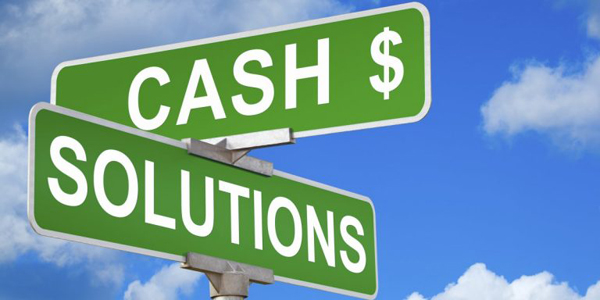Home / Money Blog

## How much will I owe?Note: You can use any financial calculator to do this problem, but if you want the BEST, you can get our 10bii Financial Calculator for iOS, Android, Mac, and Windows!THE SCENARIO Sometimes when you buy property, you finance the purchase with a quick, expensive loan (oftentimes called a 'hard money' loan) and then refinance it after a few months have passed (which lenders call a 'seasoning' period) to a slower-to-acquire, lower-rate loan. Hard money loans often carry a high interest rate, additional 'points'*, and generally have a short maximum lifetime - often one year or less. Some hard money loans require monthly payments and some compound the amount owed for eventual repayment at the end. The question: If I want to borrow \$60,000 worth of hard money, at a rate of 11% plus 2 points, with all payments deferred until the end of the loan, and I repay it after 8 months, how much will I end up paying back? Assume that the points are assessed at the time of repayment and thus do not accrue interest. * Each 'point' is one percent of the loaned value that will have to be repaid in addition to the amount borrowed and the interest, though most of the time the points themselves do not accrue interest. For example, if I borrow \$75,000 and pay 1 point, that would work out to \$75,000 x 1% = \$750.
THE SOLUTION This one is pretty straightforward, and includes two parts:
1. Figure out how much will be owed on the 11% loan
2. Figure out how much will be owed on the points and add that in
First things first, make sure the calculator is using 12 Payments per Year. N: 8 (I'm going to pay the loan back after 8 months) I/YR: 11 (The loan's interest rate is 11%) PV: 60,000 (I'm borrowing \$60,000) PMT: 0 (During the life of the loan, no payments are due) FV: (This is what I'm trying to find) At the end of the 8 months, the principal owed, plus the interest compounded, will be \$64,543.78.

The loan also has 2 points, which is 2% of the amount borrowed. 2% x \$60,000 is \$1,200. Add that to the amount owed on the loan to find that I'll owe \$1,200 + \$64,543.78 = \$65,743.78.

So in 8 months, I'll end up paying nearly \$6,000 to borrow \$60,000… but if the property I'm buying makes me substantially more than \$6,000, it might be worth doing. Also, I agreed to pay 11% interest which would have cost me \$64,543.78 - \$6,000 = \$4,543.78 in interest, but I'll end up paying a total of \$65,743.78 - \$60,000 = \$5,743.78. So what was the effective interest rate that I'll really pay? Find out in the next Money Blog!

What do you think? Would you pay high rates to borrow money? If not, why not? If so, what would go into the decision whether to do so on a particular deal or not? Let us know in the comments!